# 352 Results

View
Selected filters:
• MathematicsConditions of Use:
Remix and Share
Rating

Students will explore multi-digit numbers and the relationship between ones, tens and hundreds; a digit in one place is 10x the digit in the place to its right. Students will use their bodies to represent digits in multi-digit numbers up to the hundredths place and compare these numbers using <, =, >. Students will use their bodies as multi-digit numbers to add and subtract.

Material Type:
Activity/Lab
Teaching/Learning Strategy
Author:
01/28/2016Rating

This lesson unit is intended to help teachers assess how well students are able to visualize two-dimensional cross-sections of representations of three-dimensional objects. In particular, the lesson will help you identify and help students who have difficulties recognizing and drawing two-dimensional cross-sections at different points along a plane of a representation of a three-dimensional object.

Material Type:
Assessment
Lesson Plan
Provider:
Shell Center for Mathematical Education
U.C. Berkeley
Provider Set:
Mathematics Assessment Project (MAP)
04/26/2013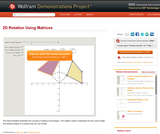Conditions of Use:
Remix and Share
Rating

This Demonstration illustrates the concept of rotating a 2D polygon. The rotation matrix is displayed for the current angle. The default polygon is a square that you can modify.

Material Type:
Activity/Lab
Diagram/Illustration
Homework/Assignment
Interactive
Simulation
Teaching/Learning Strategy
Provider:
Wolfram Research
Provider Set:
Wolfram Demonstrations Project
Author:
Mito Are and Valeria Antohe
09/04/2013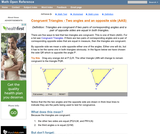Conditions of Use:
Rating

An interactive applet and associated web page that shows how triangles that have two angles and a non-included side the same must be congruent. The applet shows two triangles, one of which can be reshaped by dragging any vertex. The other changes to remain congruent to it and the two angles and non-included side are outlined in bold to show they are the same measure and are the elements being used to prove congruence. The web page describes all this and has links to other related pages. Applet can be enlarged to full screen size for use with a classroom projector. This resource is a component of the Math Open Reference Interactive Geometry textbook project at http://www.mathopenref.com.

Material Type:
Simulation
Provider:
Math Open Reference
Author:
John Page
02/16/2011Conditions of Use:
No Strings Attached
Rating

This task examines the ways in which the plane can be covered by regular polygons in a very strict arrangement called a regular tessellation. These tessellations are studied here using algebra, which enters the picture via the formula for the measure of the interior angles of a regular polygon (which should therefore be introduced or reviewed before beginning the task). The goal of the task is to use algebra in order to understand which tessellations of the plane with regular polygons are possible.

Material Type:
Activity/Lab
Provider:
Illustrative Mathematics
Provider Set:
Illustrative Mathematics
Author:
Illustrative Mathematics
01/21/2013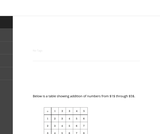Conditions of Use:
No Strings Attached
Rating

The purpose of this task is to study some patterns in a small addition table. Each pattern identified persists for a larger table and if more time is available for this activity students should be encouraged to explore these patterns in larger tables.

Material Type:
Activity/Lab
Provider:
Illustrative Mathematics
Provider Set:
Illustrative Mathematics
Author:
Illustrative Mathematics
08/15/2012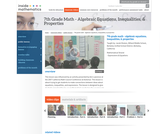Conditions of Use:
Rating

This lesson is about trying to get students to make connections between ideas about equations, inequalities, and expressions. The lesson is designed to give students opportunities to use mathematical vocabulary for a purpose to describe, discuss, and work with these symbol strings.The idea is for students to start gathering global information by looking at the whole number string rather than thinking only about individual procedures or steps. Hopefully students will begin to see the symbol strings as mathematical objects with their own unique set of attributes. (7th Grade Math)

Material Type:
Activity/Lab
Lecture
Lesson Plan
Teaching/Learning Strategy
Provider:
Noyce Foundation
Provider Set:
Inside Mathematics
Author:
Disston, Jacob
11/30/2011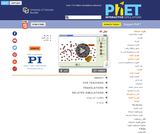Conditions of Use:
قراءة النص المطبوع بخط رفيع
Rating

شاهد هروب جسيمات ألفا من نواة البولونيوم، مسببة تحلل ألفا المشعة. شاهد كيف أن عدد مرات التسوس العشوائي يرتبط بنصف العمر.

نوع المادة:
محاكاة
Provider:
Provider Set:
المحاكاة التفاعلية لموقع PhET
المؤلف:
Carl Wieman
Danielle Harlow
John Blanco
Kathy Perkins
Noah Podolefsky
Ron LeMaster
Sam McKagan
09/02/2012Rating

This lesson unit is intended to help teachers assess how well students are able to: work with concepts of congruency and similarity, including identifying corresponding sides and corresponding angles within and between triangles; Identify and understand the significance of a counter-example; Prove, and evaluate proofs in a geometric context.

Material Type:
Assessment
Lesson Plan
Provider:
Shell Center for Mathematical Education
U.C. Berkeley
Provider Set:
Mathematics Assessment Project (MAP)
04/26/2013Conditions of Use:
Remix and Share
Rating

In this activity, learners use a hand-made protractor to measure angles they find in playground equipment. Learners will observe that angle measurements do not change with distance, because they are distance invariant, or constant. Note: The "Pocket Protractor" activity should be done ahead as a separate activity (see related resource), but a standard protractor can be used as a substitute.

Material Type:
Activity/Lab
Provider:
Exploratorium
Author:
Exploratorium
Gordon and Betty Moore Foundation
National Science Foundation
The Exploratorium
12/07/2010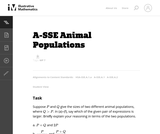Conditions of Use:
No Strings Attached
Rating

In this task students have to interpret expressions involving two variables in the context of a real world situation. All given expressions can be interpreted as quantities that one might study when looking at two animal populations.

Material Type:
Activity/Lab
Provider:
Illustrative Mathematics
Provider Set:
Illustrative Mathematics
Author:
Illustrative Mathematics
05/01/2012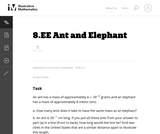Conditions of Use:
No Strings Attached
Rating

In this problem students are comparing a very small quantity with a very large quantity using the metric system. The metric system is especially convenient when comparing measurements using scientific notations since different units within the system are related by powers of ten.

Material Type:
Activity/Lab
Provider:
Illustrative Mathematics
Provider Set:
Illustrative Mathematics
Author:
Illustrative Mathematics
05/01/2012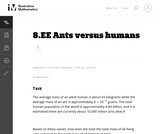Conditions of Use:
No Strings Attached
Rating

This task requires students to work with very large and small values expressed both in scientific notation and in decimal notation (standard form). In addition, students need to convert units of mass.

Material Type:
Activity/Lab
Provider:
Illustrative Mathematics
Provider Set:
Illustrative Mathematics
Author:
Illustrative Mathematics
08/21/2012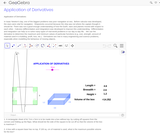Conditions of Use:
Remix and Share
Rating

We use the derivative to determine the maximum and minimum values of particular functions (e.g. cost, strength, amount of material used in a building, profit, loss, etc.).Differentiation is also used in analysis of finance and economics.

Material Type:
Simulation
Provider:
GeoGebra
Provider Set:
GeoGebraTube
03/12/2012Rating

This lesson unit is intended to help you assess how well students are able to use geometric properties to solve problems. In particular, it will support you in identifying and helping students who have the following difficulties: Solving problems relating to using the measures of the interior angles of polygons; and solving problems relating to using the measures of the exterior angles of polygons.

Material Type:
Assessment
Lesson Plan
Provider:
Shell Center for Mathematical Education
U.C. Berkeley
Provider Set:
Mathematics Assessment Project (MAP)
04/26/2013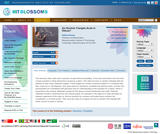Conditions of Use:
Remix and Share
Rating

This learning video deals with a question of geometrical probability. A key idea presented is the fact that a linear equation in three dimensions produces a plane. The video focuses on random triangles that are defined by their three respective angles. These angles are chosen randomly subject to a constraint that they must sum to 180 degrees. An example of the types of in-class activities for between segments of the video is: Ask six students for numbers and make those numbers the coordinates x,y of three points. Then have the class try to figure out how to decide if the triangle with those corners is acute or obtuse.

Material Type:
Lecture
Provider:
MIT
Provider Set:
MIT Blossoms
Author:
Gilbert Strange
06/02/2012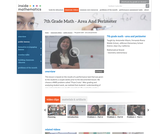Conditions of Use:
Rating

This lesson is based on the results of a performance task in which we realized that students' understanding of area and perimeter was mostly procedural. Therefore the purpose of this re-engagement lesson was to address student misconceptions and deepen student understanding of area and perimeter. The standards addressed in this lesson involve finding perimeter and area of various shapes, finding the perimeter when given a fixed area, and using a formula in a practical context. Challenges for our students included decoding the language in the problem and proving their thinking. (7th Grade Math)

Material Type:
Activity/Lab
Lecture
Lesson Plan
Teaching/Learning Strategy
Provider:
Noyce Foundation
Provider Set:
Inside Mathematics
Author:
Villarin, Antoinette
11/30/2011Conditions of Use:
Rating

Remember your multiplication tables? ... me neither. Brush up on your multiplication, division, and factoring skills with this exciting game. No calculators allowed!

Material Type:
Simulation
Provider:
Provider Set:
PhET Interactive Simulations
Author:
Michael Dubson
Trish Loeblein
05/02/2010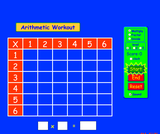Conditions of Use:
No Strings Attached
Rating

Brush up on your multiplication, division, and factoring skills with this interactive multiplication chart. Three levels and timed or untimed options are available.

Material Type:
Simulation
Provider: